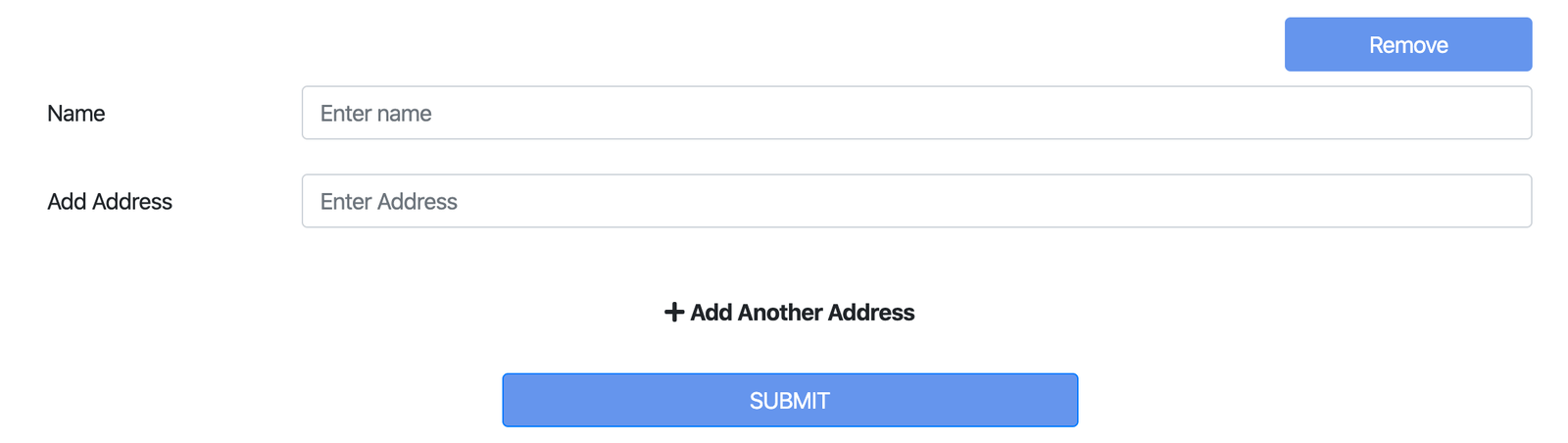Write an article or Share a link

# jQuery each() Function with Examples

1 year agoIn this Article, You will learn How to use the jQuery .each() Function (with Examples). Also, I have validate form by using .each() in JQuery. The jQuery each() function which will allow us to loop through different dataset such as arrays or objects or you can say, The each() method specifies a function to run for each matched element.

Also, we will build Minor Project using .each(). Where user can Add Remove Multiple Input Fields Using Jquery With Validation.For Demo & Source Code Scroll down.

#Syntax to use .each()
```              ```
\$(selector).each(function(index,element))
```
```

Where, function(index,element) -

• 1. index - The index position of the selector
• 2. element - The current element

When .each() called it iterates & run for each matched element that are part of the jQuery object. It is passed the current loop iteration from 0.

#Example 1

Alert the text of each `<div>` element:

```              ```
<div class="example">hello</div>
<div class="example">world</div>
```
```

By using JQuery, .each() select the `<div>` items and iterate across them:

```              ```
\$(".example").each(function(index) {
alert(index + ': ' + \$(this).text());
});
```
```

Now, output would be:

• 0: hello
• 1: world

Where, 0,1 is the index value of text in `<div>` element. Which will display in alert when you will run the above code.

#Example 2

If you want to stop loop, You can use return false.

```              ```
\$(".example").each(function(index) {
alert(index + ': ' + \$(this).text());
return false;
});
```
```

In this loop will exit after one iteration, Output of above code would be:

• 0: hello
#Lets build minor project!

After, understanding basic oh .each(), you are all set to build minor project. Where user can Add Remove Multiple Input Fields Using Jquery With Validation. Lets start building this.Add Remove Multiple Form Project Image

In above project, User can Add Multiple Address by clicking on Add Another Address. Where as Remove button is for remove multiple form but it will only work when there is more than One Form otherwise it will display alert One Address filed is compulsory.

#Lets go through from JS File!

Here is the all view of working JS code for both Add Remove Multiple Input Fields Using Jquery & With Validation

```                ```
\$(".pre-field-wrapper").each(function() {
var wrapper = \$(".pre-fields", this);
\$(".pre-field:first-child", wrapper)
.clone(true)
.appendTo(wrapper)
.find("input")
.val("")
.next()
.hide()
.focus();
});
\$(".pre-field .remove-pre-field", wrapper).click(function() {
if (\$(".pre-field", wrapper).length > 1) {
\$(this)
.parents(".pre-field")
.remove();
} else {
}
});
});

//Validation
\$("#my_form").on("submit", function() {
var form_errors = false;
if (\$.trim(\$(this).val()).length == 0) {
\$(this)
.next()
.show();
\$(this).focus();
form_errors = true;
return false;
} else {
\$(this)
.next()
.hide();
}
});
}
if (form_errors == true) {
return false;
} else {
For validation, The .each() will iterate on Class name `.addComponent` where it will match that those Input tag which have `.addComponent` class will validate on various conditions in above code. Where i have validate form on empty value basis.